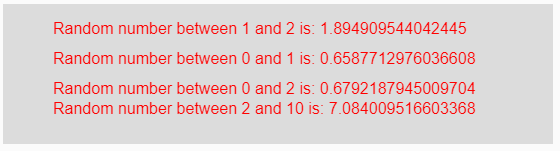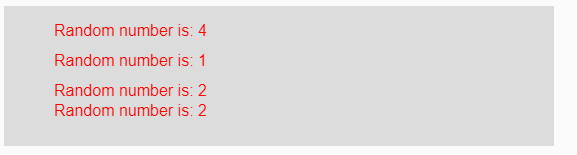# p5.js | random() Function

The random() function in p5.js is used to return a random floating point number between ranges given as the parameter.

Syntax:

`random(Min, Max)`

or

`random(Array)`

Parameters: This function accepts three parameters as mentioned above and described below:

• Min: This is the lower bound of the random number which is going to be created. This is an inclusive number with the created random number.
• Max: This is the upper bound of the random number which is going to be created. This is an exclusive number with the created random number.
• Array: This is an array of some elements from which any random number is returned.

Return Value: It returns the random number.

Below programs illustrate the random() function in p5.js:

Example 1: This example uses random() function to return a random floating point number between the given range.

 `function` `setup() {  ` `  `  `    ``// Creating Canvas size ` `    ``createCanvas(550, 140);  ` `      `  `    ``// Set the background color  ` `    ``background(220);  ` `     `  `    ``// Calling to random() function with ` `    ``// min and max parameters ` `    ``let A = random(1, 2); ` `    ``let B = random(0, 1); ` `    ``let C = random(2); ` `    ``let D = random(2, 10); ` `     `  `    ``// Set the size of text  ` `    ``textSize(16);  ` `      `  `    ``// Set the text color  ` `    ``fill(color(``'red'``));  ` `    `  `    ``// Getting random number ` `    ``text(``"Random number between 1 and 2 is: "` `+ A, 50, 30); ` `    ``text(``"Random number between 0 and 1 is: "` `+ B, 50, 60); ` `    ``text(``"Random number between 0 and 2 is: "` `+ C, 50, 90); ` `    ``text(``"Random number between 2 and 10 is: "` `+ D, 50, 110); ` `}  `

Output:Note: In the above code, in variable “C” only one parameter is passed then it returns a random number from lower bound 0 to upper bound of that number.

Example 2: This example uses random() function to return a random floating point number between the given range.

 `function` `setup() {  ` `  `  `    ``// Creating Canvas size ` `    ``createCanvas(550, 140);  ` `      `  `    ``// Set the background color  ` `    ``background(220);  ` `     `  `    ``// Calling to random() function with ` `    ``// parameter array of some elements ` `    ``let A = random([1, 2, 3, 4]); ` `    ``let B = random([0, 1]); ` `    ``let C = random([2, 6, 7, 9]); ` `    ``let D = random([2, 10]); ` `     `  `    ``// Set the size of text  ` `    ``textSize(16);  ` `      `  `    ``// Set the text color  ` `    ``fill(color(``'red'``));  ` `    `  `    ``// Getting random number ` `    ``text(``"Random number is: "` `+ A, 50, 30); ` `    ``text(``"Random number is: "` `+ B, 50, 60); ` `    ``text(``"Random number is: "` `+ C, 50, 90); ` `    ``text(``"Random number is: "` `+ D, 50, 110); ` `}  `

Output:Reference: https://p5js.org/reference/#/p5/random

My Personal Notes arrow_drop_upCheck out this Author's contributed articles.

If you like GeeksforGeeks and would like to contribute, you can also write an article using contribute.geeksforgeeks.org or mail your article to contribute@geeksforgeeks.org. See your article appearing on the GeeksforGeeks main page and help other Geeks.

Please Improve this article if you find anything incorrect by clicking on the "Improve Article" button below.

Article Tags :

Be the First to upvote.

Please write to us at contribute@geeksforgeeks.org to report any issue with the above content.